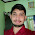# MATLAB Program for the Generation of Circular Convolution for the given Sequences | IT1254 - DSP and Communications Systems Lab

AIM:
To write a program for the generation of Circular convolution for the given sequence by using MATLAB in IT1254 - DSP and Communications Systems Lab.

ALGORITHM:
Step 1: Start
Step 2: Read the first sequence
Step 3: Read the second sequence
Step 4: Find the length of first sequence
Step 5: Find the length of second sequence
Step 6: Perform circular convolution for both the sequences
Step 7: Plot the sequence
Step 8: Display the output sequence
Step 9: Stop

PROGRAM:
clc;
g=input('Enter the sequence 1:');
h=input('Enter the sequence 2:');
N1=length(g);
N2=length(h);
N=max(N1,N2);
N3=N1-N2;
if(N3>0)
h=[h,zeros(1,N3)];
else
g=[g,zeros(1,-N3)];
end
for n=1:N;
y(n)=0;
for i=1:N;
j=n-i+1;
if(j<=0)
j=N+j;
end
y(n)=[y(n)+(g(i)*h(j))];
end
end
disp('The resultant is');y
subplot(2,1,1);
stem(y);
xlabel('N->');
ylabel('Amplititude->');
OUTPUT:Click to view full size image!

RESULT:
Thus the generation of Circular convolution was performed using MATLAB and the output sequences were drawn.
Previous
Next Post »

Anonymous
AUTHOR

Starting with the name of ALLAH,
Assalam O Alaikum
Respected Brother, Your blog is very useful for me. With the bless of ALLAH my problem regarding circular convolution is solved. Thanks May ALLAH guide us Ameen.